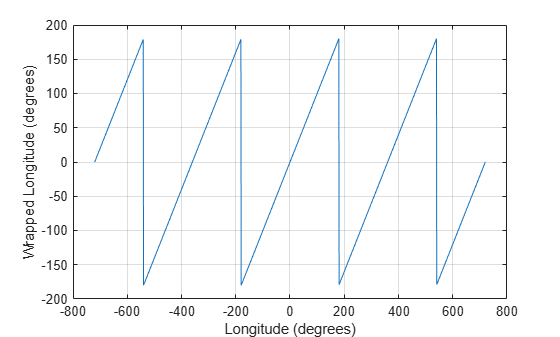# wrapTo180

Wrap angle in degrees to [-180 180]

## Syntax

``lonWrapped = wrapTo180(lon)``

## Description

example

````lonWrapped = wrapTo180(lon)` wraps angles in `lon`, in degrees, to the interval [–180, 180] such that 180 maps to 180 and –180 maps to –180. In general, odd, positive multiples of 180 map to 180 and odd, negative multiples of 180 map to –180.```

## Examples

collapse all

Specify a short list of longitudes to wrap.

`lon = [-400 -190 -180 -175 175 180 190 380];`

Wrap the longitudes to the range [-180, 180] degrees.

`lonWrapped = wrapTo180(lon)`
```lonWrapped = 1×8 -40 170 -180 -175 175 180 -170 20 ```

Specify a second list of longitudes that are sampled over a large range of angles. Wrap the longitudes.

```lon2 = -720:720; lon2Wrapped = wrapTo180(lon2);```

Plot the wrapped longitudes. The wrapped longitudes stay in the range [-180, 180] degrees.

```plot(lon2,lon2Wrapped) xlabel("Longitude (degrees)") ylabel("Wrapped Longitude (degrees)") grid on```## Input Arguments

collapse all

Angles, specified as a numeric vector.

Data Types: `single` | `double` | `int8` | `int16` | `int32` | `int64` | `uint8` | `uint16` | `uint32` | `uint64` | `logical`

## Output Arguments

collapse all

Wrapped angles, specified as a numeric vector with values in the range [–180, 180].

## Extended Capabilities

### C/C++ Code GenerationGenerate C and C++ code using MATLAB® Coder™.